Home > CC4 > Chapter A > Lesson A.1.5 > ProblemA-53

A-53.

A-53. Simplify each expression below as much as possible. Record your work in the same way you did in problem A‑51. Homework Help ✎

1. 3y − y + 5x + 3 − 7x

2. −1 − (−5x) − 2x + 2x2 + 7

3. 6x + 2 − 1 − 4x − 3 − 2x + 2

4.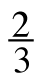x − 3y +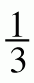x + 2y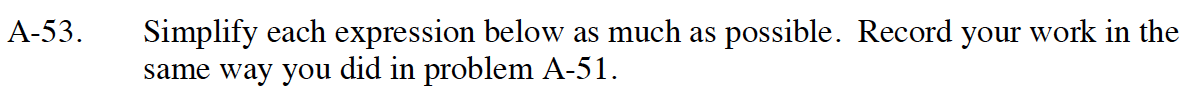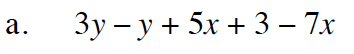Flip subtraction to addition.

3y + −y + 5x + 3 + −7x

Remove zero pairs.

2y + −2x + 3

Remember to remove unnecessary signs (Example: x + −2 would be x − 2).

2y − 2x + 3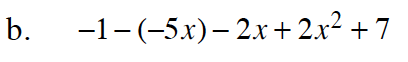Follow the steps described in part (a).

Remember a negative multiplied by a negative is a positive.

Remember you cannot add variables with different exponent values. (Example: you cannot add x + x²)

2x2 + 3x +6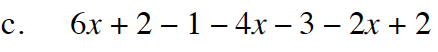Follow the steps explained inpart (a) and remember the hints in part (b).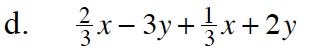$\frac{2}{3}\textit{x}+\frac{1}{3}\textit{x}\ =\ \textit{x}$